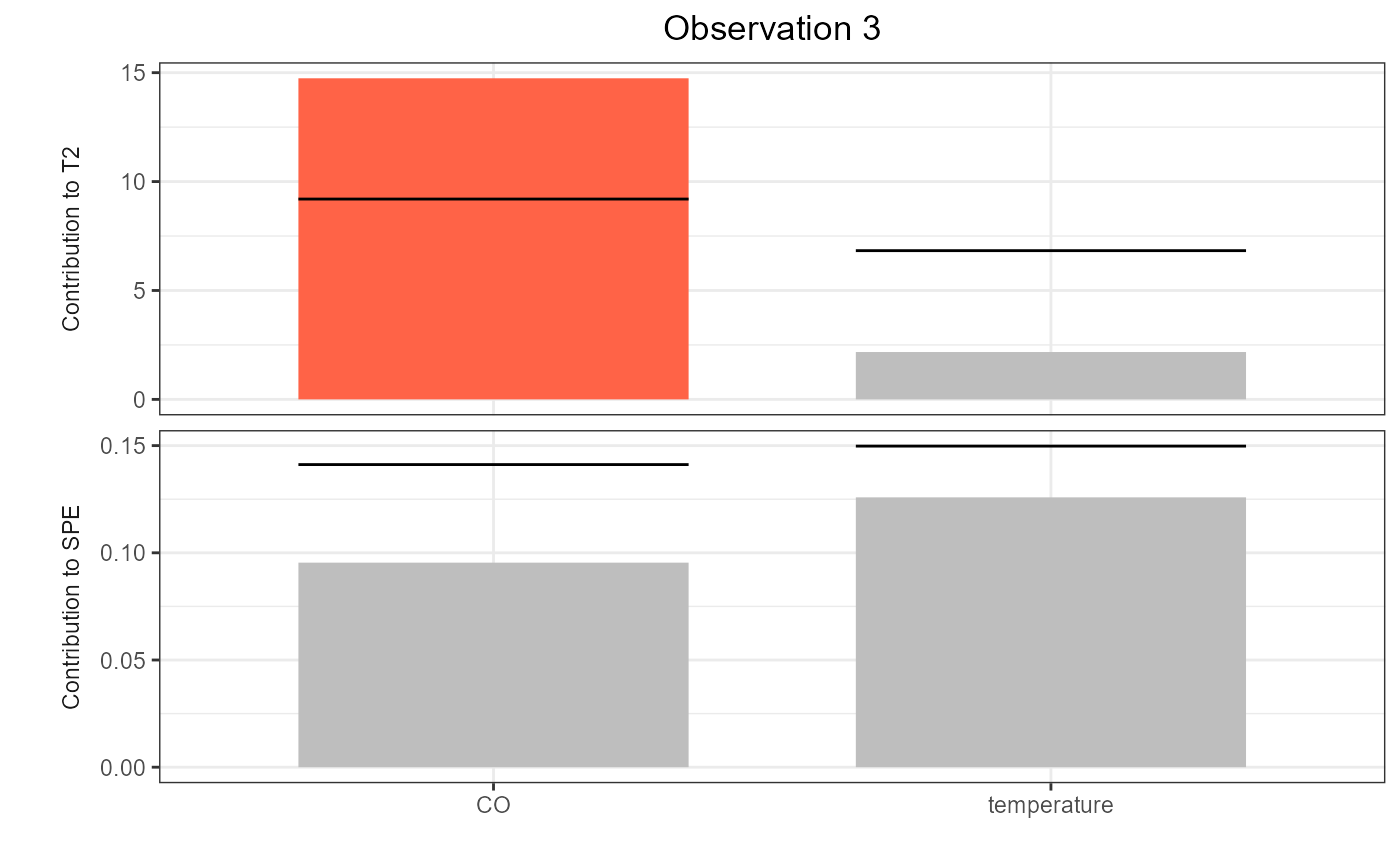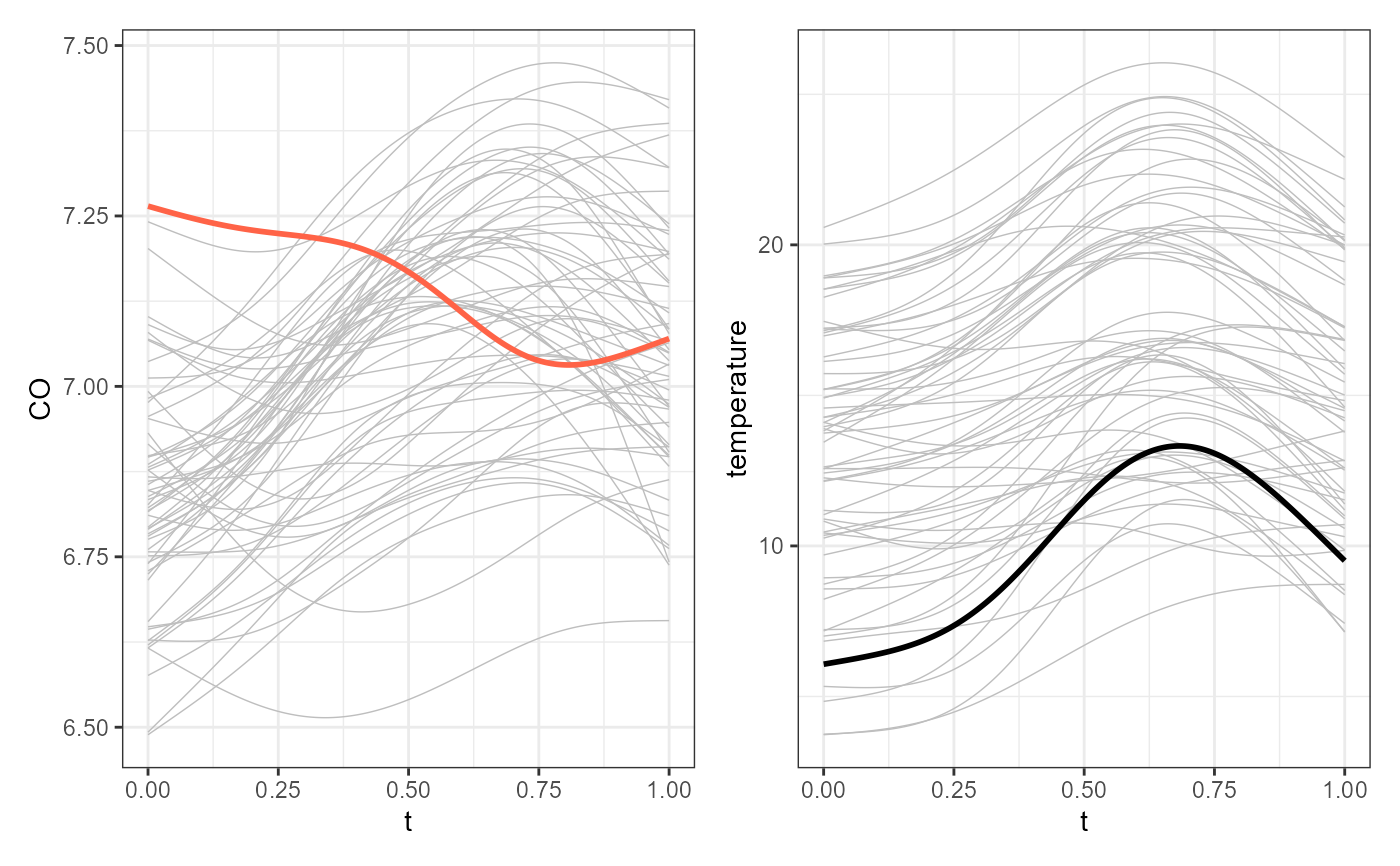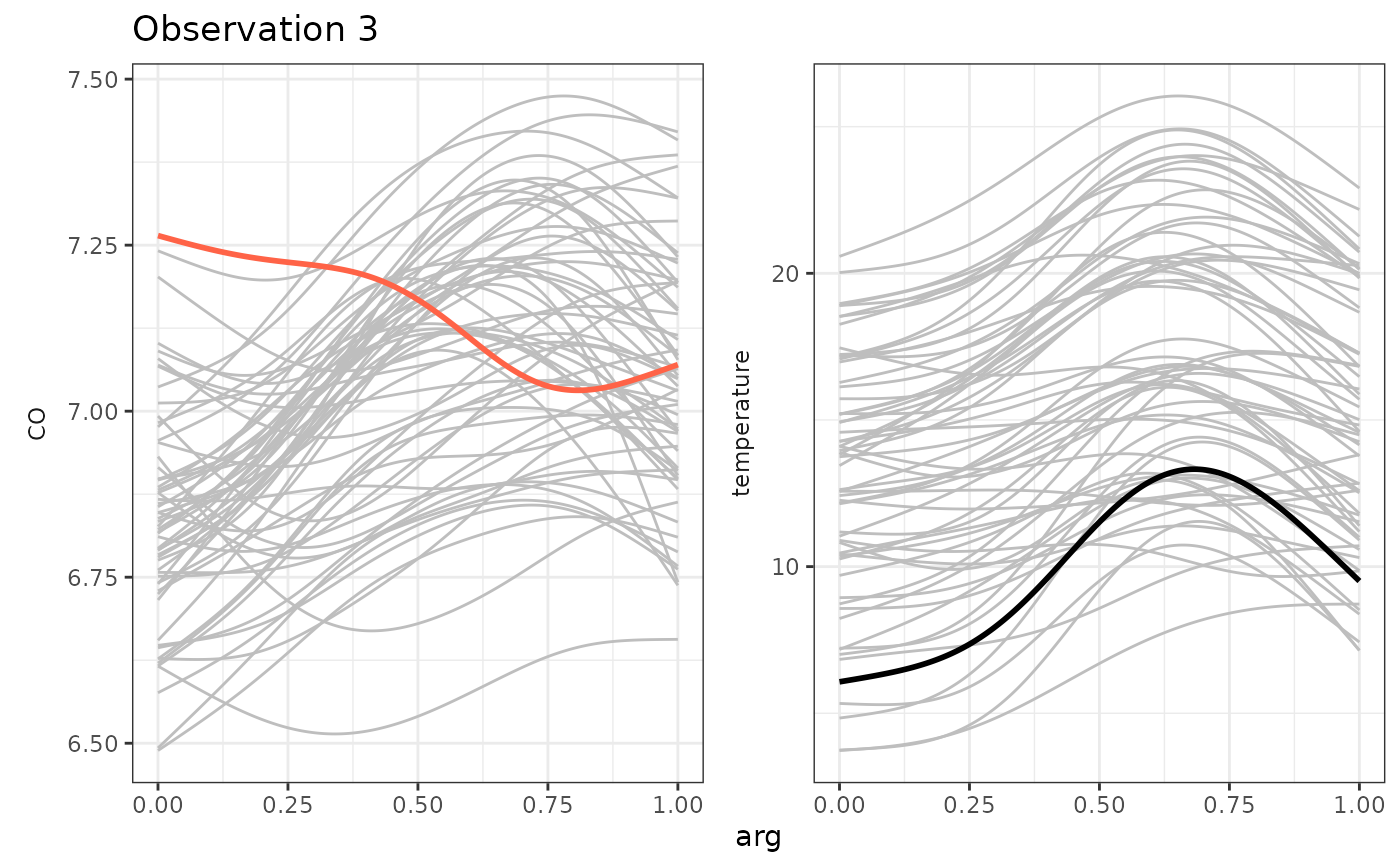This function plots selected functions in a phase_II monitoring data set against the corresponding training data set to be compared.

plot_mon(cclist, fd_train, fd_test, print_id = FALSE)

## Arguments

cclist

A data.frame produced by control_charts_pca, control_charts_sof_pc regr_cc_fof, or regr_cc_sof.

fd_train

An object of class mfd containing the training data set of the functional variables. They are plotted in gray in the background.

fd_test

An object of class mfd containing the phase II data set of the functional variables to be monitored. They are coloured in black or red on the foreground.

print_id

A logical value, if TRUE, it prints also the id of the observation in the title of the ggplot.

## Value

A ggplot of the multivariate functional data. In particular, the multivariate functional data given in fd_train are plotted on the background in gray, while the multivariate functional data given in fd_test are plotted on the foreground, the colour of each curve is black or red depending on if that curve was signal as anomalous by at least a contribution plot.

## Examples

library(funcharts)
data("air")
air <- lapply(air, function(x) x[201:300, , drop = FALSE])
fun_covariates <- c("CO", "temperature")
mfdobj_x <- get_mfd_list(air[fun_covariates],
n_basis = 15,
lambda = 1e-2)
y <- rowMeans(air\$NO2)
y1 <- y[1:60]
y2 <- y[91:100]
mfdobj_x1 <- mfdobj_x[1:60]
mfdobj_x_tuning <- mfdobj_x[61:90]
mfdobj_x2 <- mfdobj_x[91:100]
mod <- sof_pc(y1, mfdobj_x1)
cclist <- control_charts_sof_pc(mod = mod,
y_test = y2,
mfdobj_x_test = mfdobj_x2,
mfdobj_x_tuning = mfdobj_x_tuning)
plot_control_charts(cclist)cont_plot(cclist, 3)plot_mon(cclist, fd_train = mfdobj_x1, fd_test = mfdobj_x2)